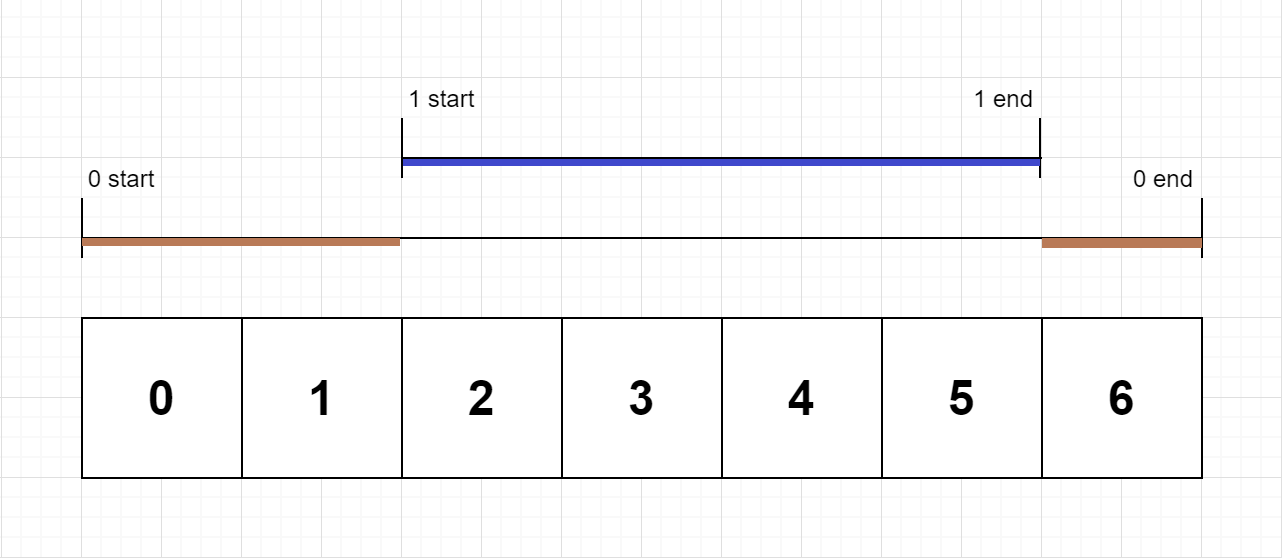0636. Exclusive Time of Functions

# 636. Exclusive Time of Functions#

## 题目 #

On a single threaded CPU, we execute some functions. Each function has a unique id between `0` and `N-1`.

We store logs in timestamp order that describe when a function is entered or exited.

Each log is a string with this format: `"{function_id}:{"start" | "end"}:{timestamp}"`. For example, `"0:start:3"` means the function with id `0` started at the beginning of timestamp `3``"1🔚2"` means the function with id `1` ended at the end of timestamp `2`.

A function’s exclusive time is the number of units of time spent in this function. Note that this does not include any recursive calls to child functions.

Return the exclusive time of each function, sorted by their function id.

Example 1:``````Input:
n = 2
logs = ["0:start:0","1:start:2","1🔚5","0🔚6"]
Output: [3, 4]
Explanation:
Function 0 starts at the beginning of time 0, then it executes 2 units of time and reaches the end of time 1.
Now function 1 starts at the beginning of time 2, executes 4 units of time and ends at time 5.
Function 0 is running again at the beginning of time 6, and also ends at the end of time 6, thus executing for 1 unit of time.
So function 0 spends 2 + 1 = 3 units of total time executing, and function 1 spends 4 units of total time executing.
``````

Note:

1. `1 <= n <= 100`
2. Two functions won’t start or end at the same time.
3. Functions will always log when they exit.

## 解题思路 #

• 利用栈记录每一个开始了但是未完成的任务，完成以后任务就 pop 一个。
• 注意题目中关于任务时长的定义，例如，start 7，end 7，这个任务执行了 1 秒而不是 0 秒

## 代码 #

``````
package leetcode

import (
"strconv"
"strings"
)

type log struct {
id    int
order string
time  int
}

func exclusiveTime(n int, logs []string) []int {
res, lastLog, stack := make([]int, n), log{id: -1, order: "", time: 0}, []log{}
for i := 0; i < len(logs); i++ {
a := strings.Split(logs[i], ":")
id, _ := strconv.Atoi(a)
time, _ := strconv.Atoi(a)

if (lastLog.order == "start" && a == "start") || (lastLog.order == "start" && a == "end") {
res[lastLog.id] += time - lastLog.time
if a == "end" {
res[lastLog.id]++
}
}
if lastLog.order == "end" && a == "end" {
res[id] += time - lastLog.time
}
if lastLog.order == "end" && a == "start" && len(stack) != 0 {
res[stack[len(stack)-1].id] += time - lastLog.time - 1
}
if a == "start" {
stack = append(stack, log{id: id, order: a, time: time})
} else {
stack = stack[:len(stack)-1]
}
lastLog = log{id: id, order: a, time: time}
}
return res
}

``````Apr 8, 2023Edit this page